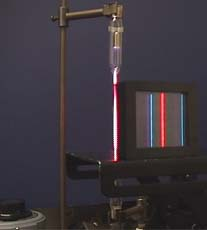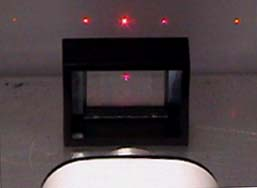# Diffraction Gratings### Reflection Diffraction Gratings

(not on the syllabus!)

### Transmission Diffraction Gratings

A transmission diffraction grating is a slide with large number of parallel, closely spaced slits (transparent spaces) drawn on it. Early ones were carbon covered glass slides etched by a needle point - now they tend to be printed onto a slide.When a parallel beam of monochromatic light is directed normally (at right angles to it!) at a diffraction grating, light is transmitted by the grating in certain directions only.

This is because:

• the light passing through each slit is diffracted,
• the diffracted light waves from adjacent slits reinforce each other in certain directions only, including the incident light direction, and cancel out in all other directions.

It is excellent at separating the colors in incident light because different wavelengths are diffracted at different angles, according to the grating relationship:

# d sin θ = nλwhere

• d is the distance between the slits
• θ is the angle of diffraction
• λ is the wavelength of the light
• n is the order of diffraction

The separation being so small makes the angle large.Consider a magnified view of part of a diffraction grating - look at the diagram on the left..

Each slit diffracts the light waves that pass through it. The diffracted waves then interfere with each other. Where crests meet or troughs meet there is reinforcement (constructive interference) – where a crest and tough meet there is cancellation (destructive interference). This results in only the ‘orders’ of light being viewed.

As each diffracted wavefront emerges from a slit, it reinforces a wavefront from each of the adjacent slits.

For example, in the diagram, the wavefront emerging at P reinforces the wavefront emitted from Q one cycle earlier, which reinforces the wavefront emitted from R one cycle earlier, etc.

The effect is to form a new wavefront PYZ which travels in a certain direction and contributes to the first order diffracted beam.The diagram on the right shows the formation of a wavefront of the nth order beam.

The wavefront emerging from slit P reinforces a wavefront emitted n cycles earlier by the adjacent slit Q.

This earlier wavefront therefore must have travelled a distance of n wavelengths from the slit.

Therefore the perpendicular distance QY from the slit to the wavefront is equal to nλ , where λ is the wavelength of the light waves.

Since the angle of diffraction of the beam,, is equal to the angle between the wavefront and the plane of the slits, it follows that sin= QY/QI where QP is the grating spacing (i.e. the centre-to-centre distance d between adjacent slits).

Substituting d for QP and nd for QY therefore gives sin= nλ /d.

Rearranging this equation gives the diffraction grating equation for the angle of diffraction of the nth order beam

# dsin θ = n λ

• The number of slits per metre on the grating, N = 1/ d where d is the grating spacing.
• For a given order and wavelength, the smaller the value of d, the greater the angle of diffraction. In other words, the larger the number of slits per metre, the bigger the angle of diffraction.
• Fractions of a degree are usually expressed either as a decimal or in minutes (abbreviated ') where 1° = 60'.
• To find the maximum number of orders produced, substitute= 90 ° (sin= 1) in the grating equation and calculate n using n = d/.

The maximum number of orders is given by the value of d/, rounded down to the nearest whole number.

-----------------------------------------------

The diffraction grating is an immensely useful tool for the separation of the spectral lines associated with atomic transitions. It separates the different colors of light much more than the dispersion effect in a prism is able to - it uses diffraction not refraction to do it!Note that from the equation you can see that, the bigger the wavelength the more diffraction. This means that it is the red end of the visible spectrum that is diffracted the most. In the prism the red end of the spectrum is refracted the least!

See here for a practical investigation you can carry out using a diffraction grating.The illustration shows the hydrogen spectrum. The hydrogen gas in a thin glass tube is excited by an electrical discharge, the electrons are promoted by electrical energy into higher energy states - an then as they fall back to ground state some of the transitions result in photons in the visible region being emitted. This visible spectrum can be viewed through the grating. Click onto the image to go to an excellent site that explains this in even more detail and allows you to explore diffraction via an interactive activity.

The condition for maximum intensity is the same as that for the double slit or multiple slits, but with a large number of slits the intensity maximum is very sharp and narrow, providing the high resolution for spectroscopic applications. The peak intensities are also much higher for the grating than for the double slit.When monochromatic light (light of a single wavelength - like the 632.8 nm red light from a helium-neon laser) strikes a diffraction grating it is diffracted to each side in multiple orders. The condition for maximum intensity is the same as that for a double slit. However, angular separation of the maxima is generally much greater because the slit spacing is so small for a diffraction grating.

• You can make a simple grating at home - see this linkNow try some multiple choice questions on diffraction.